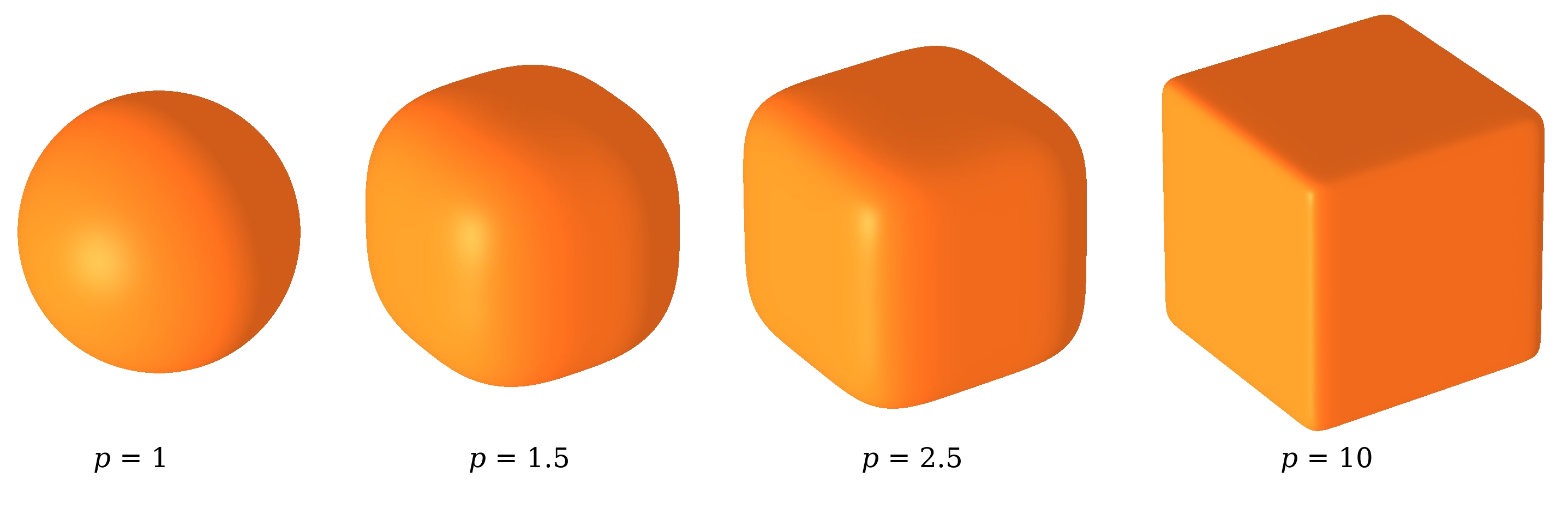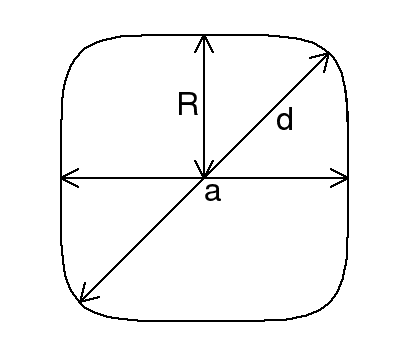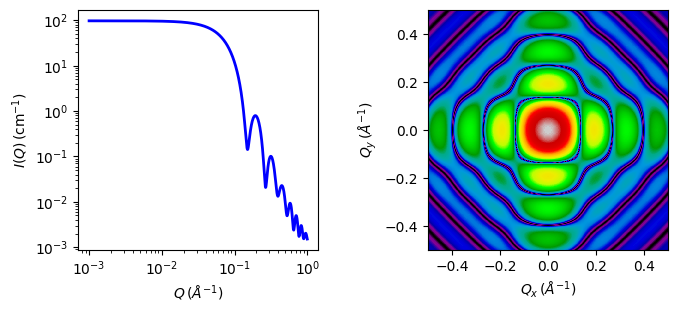# superball

Superball with uniform scattering length density.

Parameter

Description

Units

Default value

scale

Scale factor or Volume fraction

None

1

background

Source background

cm-1

0.001

sld

Superball scattering length density

10-6-2

4

sld_solvent

Solvent scattering length density

10-6-2

1

length_a

Cube edge length of the superball

50

exponent_p

Exponent describing the roundness of the superball

None

2.5

theta

c axis to beam angle

degree

0

phi

degree

0

psi

rotation about c axis

degree

0

The returned value is scaled to units of cm-1 sr-1, absolute scale.

DefinitionFig. 92 Superball visualisation for varied values of the parameter p.

This model calculates the scattering of a superball, which represents a cube with rounded edges. It can be used to describe nanoparticles that deviate from the perfect cube shape as it is often observed experimentally . The shape is described by

$x^{2p} + y^{2p} + z^{2p} \leq \biggl( \frac{a}{2} \biggr)^{2p}$

with $$a$$ the cube edge length of the superball and $$p$$ a parameter that describes the roundness of the edges. In the limiting cases $$p=1$$ the superball corresponds to a sphere with radius $$R = a/2$$ and for $$p = \infty$$ to a cube with edge length $$a$$. The exponent $$p$$ is related to $$a$$ and the face diagonal $$d$$ via

$p = \frac{1}{1 + 2 \mathrm{log}_2 (a/d)}.$Fig. 93 Cross-sectional view of a superball showing the principal axis length $$a$$, the face-diagonal $$d$$ and the superball radius $$R$$.

The oriented form factor is determined by solving

$\begin{split}p_o(\vec{q}) =& \int_{V} \mathrm{d} \vec{r} e^{i \vec{q} \cdot \vec{r}}\\ =& \frac{a^3}{8} \int_{-1}^{1} \mathrm{d} x \int_{-\gamma}^{\gamma} \mathrm{d} y \int_{-\zeta}^{\zeta} \mathrm{d} z e^{i a (q_x x + q_y y + q_z z) / 2}\\ =& \frac{a^2}{2 q_z} \int_{-1}^{1} \mathrm{d} x \int_{-\gamma}^{\gamma} \mathrm{d} y e^{i a(q_x x + q_y y)/2} \sin(q_z a \zeta / 2),\end{split}$

with

$\begin{split}\gamma =& \sqrt[2p]{1-x^{2p}}, \\ \zeta =& \sqrt[2p]{1-x^{2p} -y^{2p}}.\end{split}$

The integral can be transformed to

$p_o(\vec{q}) = \frac{2 a^2}{q_z} \int_{0}^{1} \mathrm{d} x \, \cos \biggl(\frac{a q_x x}{2} \biggr) \int_{0}^{\gamma} \mathrm{d} y \, \cos \biggl( \frac{a q_y y}{2} \biggr) \sin \biggl( \frac{a q_z \zeta}{2} \biggr),$

which can be solved numerically.

The orientational average is then obtained by calculating

$P(q) = \int_0^{\tfrac{\pi}{2}} \mathrm{d} \varphi \int_0^{\tfrac{\pi}{2}} \mathrm{d} \theta \, \sin (\theta) | p_o(\vec{q}) |^2$

with

$\begin{split}\vec{q} &= q \begin{pmatrix} \cos (\varphi) \sin (\theta)\\ \sin (\varphi) \sin(\theta)\\ \cos (\theta)\end{pmatrix}\end{split}$

The implemented orientationally averaged superball model is then fully given by 

$I(q) = \mathrm{scale} (\Delta \rho)^2 P(q) + \mathrm{background}.$

FITTING NOTES

Validation

The code is validated by reproducing the spherical form factor implemented in SasView for $$p = 1$$ and the parallelepiped form factor with $$a = b = c$$ for $$p = 1000$$. The form factors match in the first order oscillation with a precision in the order of $$10^{-4}$$. The agreement worsens for higher order oscillations and beyond the third order oscillations a higher order Gauss quadrature rule needs to be used to keep the agreement below $$10^{-3}$$. This is however avoided in this implementation to keep the computation time fast.Fig. 94 1D and 2D plots corresponding to the default parameters of the model.

Source

superball.py $$\ \star\$$ superball.c $$\ \star\$$ sas_gamma.c $$\ \star\$$ gauss20.c

References

Source

superball.py

superball.c

Authorship and Verification

• Author: Dominique Dresen Date: March 27, 2019

• Last Modified by: Dominique Dresen Date: March 27, 2019

• Last Reviewed by: Dirk Honecker Date: November 05, 2021

• Source added by : Dominique Dresen Date: March 27, 2019